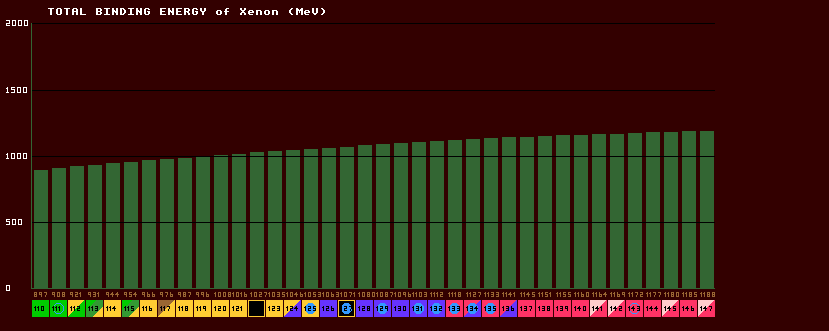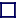### TOTAL BINDING ENERGY (BE)

(expressed in Mega electron volts, MeV)

 The mass of an isotope is not simply the sum of the masses of all the constituent nucleons (protons and neutrons) within the nucleus, because when they are all gathered together inside the nucleus, some of the mass (energy) is 'lost' and this loss is called the binding energy, which holds all the nucleons together and is responsible for the stability of the nucleus. The total binding energy rises slowly for the first few elements, but steadily increases with increasing atomic number (number of protons). Numerically, BE=931×[(neutron number × neutron mass + atomic number × proton mass) - mass defect]. Shown is the total binding energy plot for the isotopes of xenon. Note that the total binding energy is almost proportional to the atomic weight, A, because the binding energy per nucleon is almost constant at approximately 8MeV (the saturation value) for elements heavier than nitrogen.